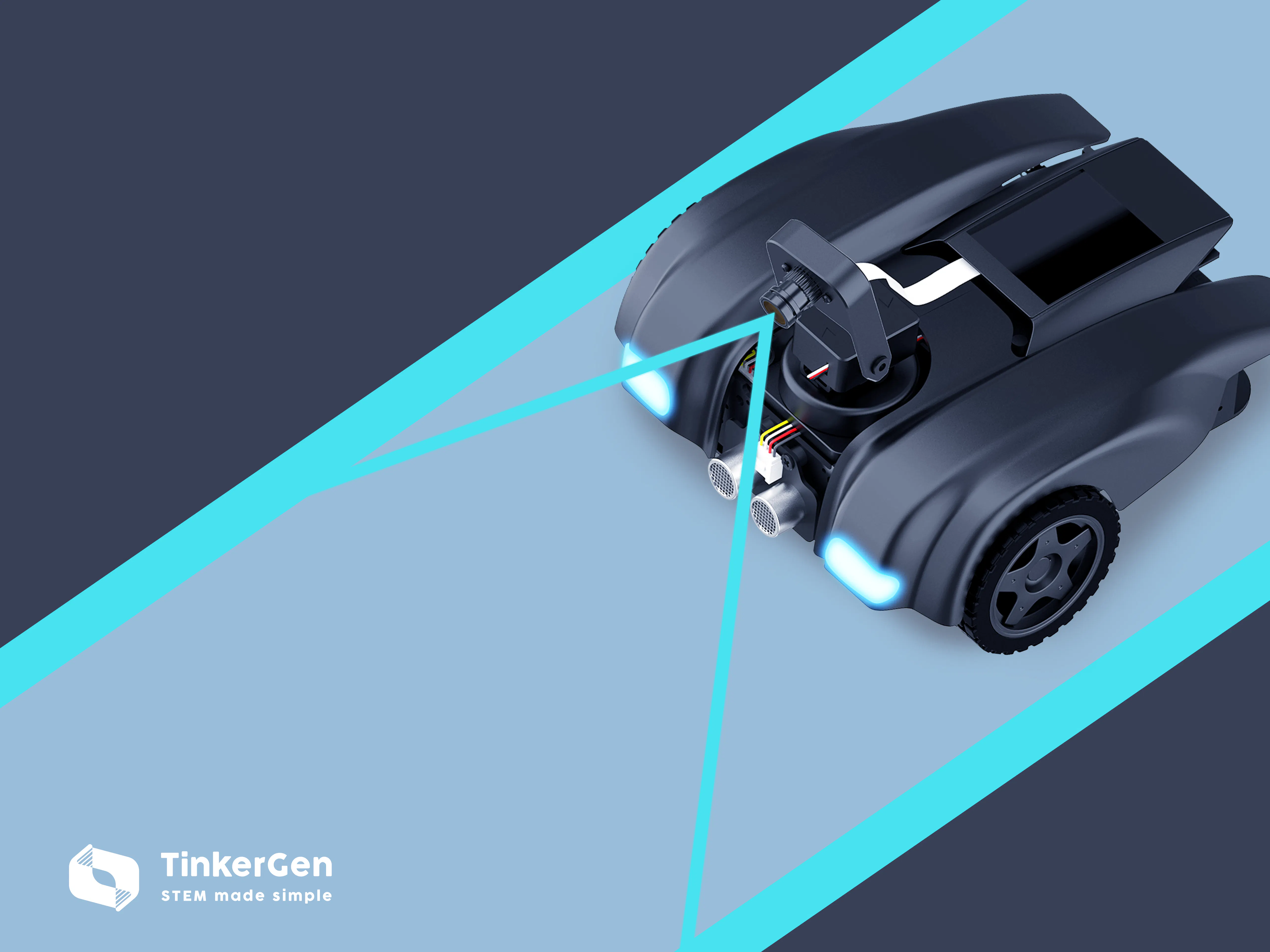# Lane Following: OpenMV and DeepLearning approaches with K210

Man vs. machine. Wait, it's actually Machine vs. Machine

IntermediateProtip6 hours748## Things used in this project

### Hardware components

 Seeed MARK
×1

### Software apps and online services

 aXeleRate

## Code

### Gathering the data

MicroPython
```import sensor, image, time, lcd, os, video
from maix_motor import Maix_motor
from gpio import *

lcd.init()
sensor.reset()                      # Reset and initialize the sensor. It will
# run automatically, call sensor.run(0) to stop
sensor.set_pixformat(sensor.RGB565) # Set pixel format to RGB565 (or GRAYSCALE)
sensor.set_framesize(sensor.QVGA)   # Set frame size to QVGA (320x240)
sensor.set_vflip(1)
clock = time.clock()                # Create a clock object to track the FPS.

v = video.open("/sd/capture.avi", record=1, interval=200000, quality=90)
f = open("/sd/capture.txt",'w')

counter = 0
tim = time.ticks_ms()

def save_img(img,count,value):
img_len = v.record(img)
f.write(str(count)+'-'+str(value)+'\n')

while(time.ticks_diff(time.ticks_ms(), tim)<1000*30):
img = sensor.snapshot()
lcd.display(img)
if DigitalIn_button(9) and DigitalIn_button(10):
save_img(img,counter,1)
Maix_motor.motor_motion(2, 1, 0)
tim = time.ticks_ms()
else:
if DigitalIn_button(9):
save_img(img,counter,4)
Maix_motor.motor_motion(2, 4, 0)

if DigitalIn_button(10):
save_img(img,counter,3)
Maix_motor.motor_motion(2, 3, 0)

if not DigitalIn_button(9) and not DigitalIn_button(10):
Maix_motor.motor_run(0, 0, 0)
counter = counter + 1

v.record_finish()
f.close()
lcd.clear()
```

### Driving with trained classifier

MicroPython
```import sensor, image, lcd, time
import KPU as kpu
from maix_motor import Maix_motor

lcd.init()
sensor.reset()
sensor.set_pixformat(sensor.RGB565)
sensor.set_framesize(sensor.QVGA)
#sensor.set_windowing((224, 224))
sensor.set_vflip(1)
lcd.clear()

labels = ['forward','left','right']

while(True):
img = sensor.snapshot()
img = img.resize(224,224)
img = img.rotation_corr(z_rotation=90.0)
a = img.pix_to_ai()
plist=fmap[:]
pmax=max(plist)
max_index=plist.index(pmax)
a = img.draw_string(0,0, str(labels[max_index].strip()), color=(255,0,0), scale=2)
a = img.draw_string(0,20, str(pmax), color=(255,0,0), scale=2)
direction = labels[max_index].strip()
if direction=='forward':
Maix_motor.motor_left(100, 0)
Maix_motor.motor_right(100, 0)
else:
if direction=='right':
Maix_motor.motor_left(40, 0)
Maix_motor.motor_right(-30, 0)

if direction=='left':
Maix_motor.motor_left(-30, 0)
Maix_motor.motor_right(40, 0)

a = lcd.display(img)

```

### OpenMV lane detection

MicroPython
```enable_recording = False

import sensor, image, time, lcd, math, video
from gpio import *
from modules import ws2812
from maix_motor import Maix_motor

lcd.init()
sensor.reset()
sensor.set_pixformat(sensor.RGB565) # grayscale is faster
sensor.set_framesize(sensor.QVGA)
sensor.set_vflip(2)
clock = time.clock()

# All lines also have `x1()`, `y1()`, `x2()`, and `y2()` methods to get their end-points
# and a `line()` method to get all the above as one 4 value tuple for `draw_line()`.
high_threshold = (70, 255)
ws2812_13 = ws2812(fm.board_info.D,5,2,3)
ws2812_13.set_led(1, (255, 255, 255))
ws2812_13.display()
ws2812_13.set_led(0, (255, 255, 255))
ws2812_13.display()
tim = time.ticks_ms()

if enable_recording:
v = video.open("/flash/capture.avi", record=1, interval=200000, quality=40)
recording_length = 1000 * 30 #30 seconds
else:
recording_length = float('inf')

while(time.ticks_diff(time.ticks_ms(), tim)<120000):
clock.tick()
img = sensor.snapshot()
#img = image.Image("/flash/test10.jpg")
img = img.rotation_corr(z_rotation=90.0)

roi=(0,120,320,240)
img = img.binary([high_threshold])
#img = img.laplacian(1, add =2, mul =2)
a = img.draw_rectangle(roi, color = (0,255,0), thickness=3)
max_left = 0
max_left_line = 0
max_right = 300
max_right_line = 0
for l in img.find_lines(roi=roi,threshold = 2000,x_stride=2, y_stride=1):
if l.theta() !=89 and l.theta() != 0:
#a = img.draw_line(l.line(), color = (0, 0, 255), thickness=3)
if l.x1() >= max_left:
max_left = l.x1()
max_left_line = l
if l.x2() <= max_right:
max_right = l.x2()
max_right_line = l
if max_right_line != 0:
a = img.draw_line(max_right_line.line(), color = (255, 0, 0), thickness=3)
line_right = max_right_line.x2()
else:
line_right = 0
if max_left_line != 0:
a = img.draw_line(max_left_line.line(), color = (255, 0, 0), thickness=3)
line_left = max_left_line.x1()
else:
line_left = 0

lane_center = (line_left+line_right)//2
print(lane_center)
print(line_left)
print(line_right)
a = img.draw_arrow(160, 240, lane_center, 120, (0,0,255), thickness=3)

deflection_angle = -math.atan((lane_center-160)/120)
deflection_angle = math.degrees(deflection_angle)//2
print(deflection_angle)
Maix_motor.motor_angle(deflection_angle, 50, 60)
lcd.display(img)
#print(img.compress_for_ide(), end="")
if enable_recording: img_len = v.record(img)
print("FPS %f" % clock.fps())

v.record_finish()
lcd.clear()
```

## Credits

### Dmitry Maslov

11 projects • 22 followers